# Topic Electrochemical Cells Do Now get 5 color

• Slides: 24Topic: Electrochemical Cells Do Now: get 5 color pencils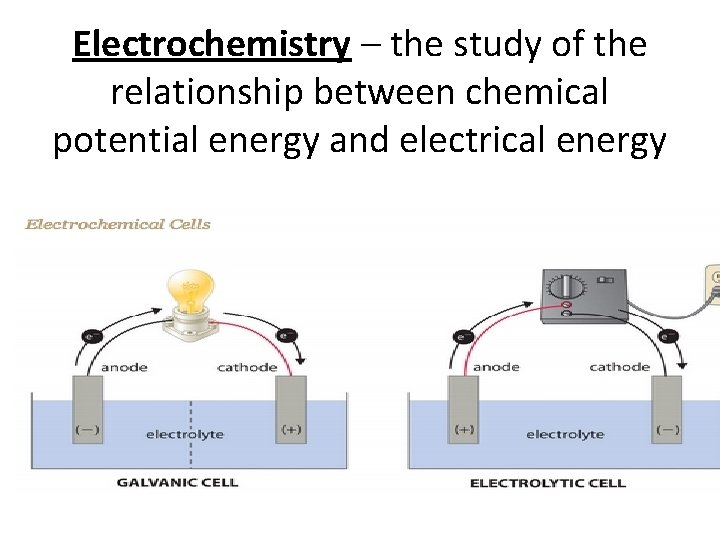Electrochemistry – the study of the relationship between chemical potential energy and electrical energy• Chemical rxns can produce electricity OR • Electrical energy can be used carry out chemical reactions All this occurs Via flow of electrons A Chemical reactions can generate electrical energy if it occurs spontaneouslyHow does a spontaneous reaction generate electricity? Electrochemical Cells AKA voltaic cell or galvanic cell • Used when the chemical rxn is spontaneous • It produces electricity (energy) via flow of electrons through a wire therefore is exothermic • Oxidation and Reduction Reaction have to be separated (half cells)Remember the Al foil lab +2 -1 0 0 +3 -1 Al. Cl 3 Cu. Cl 2 + Al _______ Cu +_______ Was this spontaneous? yes Was this a redox? Yes, Cu was Reduced & Al was Oxidized So why didn’t we produce electricity? Half reactions weren’t separatedElectrochemical Cell Requirements • 2 half-cells containing aqueous solution & an electrode • Need to be connected by a wire for the electrons to flow through. • Need to be connected by a salt bridge to maintain electrical neutrality. (if electrons are moving ions need to move also = + ions will flow in same direction as electrons) e- e- Al Cu Al+3 Cl-1 Al. Cl 3 Cl-1 Cu+2 Cu. Cl 2 -1 ClVoltmeter = measures electrical current When the cells reach equilibrium voltage = 0 =no more electrical currentElectrode Surface at which oxidation or reduction half-reaction occurs. Anode – Oxidation The anode = location for the oxidation half-reaction. Reduction – Cathode The cathode = location for the reduction half-reaction.MOVIE CLIP • MEMORIZE –Fat Red Cat ate An Ox –Anode oxidation –Fat (getting bigger) Reduction ouch Cathode yummy• Since Cu is the cathode – a fat red cat = gain mass – Where does the mass come from? – Reduction of Cu+2 is gaining 2 e- to make Cu – So the concentration of Cu+2 ions decreases • Since Al is the anode – An ox was eaten = lost e- = getting smaller – Oxidation = Lose electrons, who is going to lose Al or Al+3 – Al Al+3(aq) + 3 e– So the concentration of Al+3 increases Al Al+3 2 Al 2 Al+3(aq) + 3 e 6 - 3 Cu+2(aq) + 2 e 6 - 3 Cu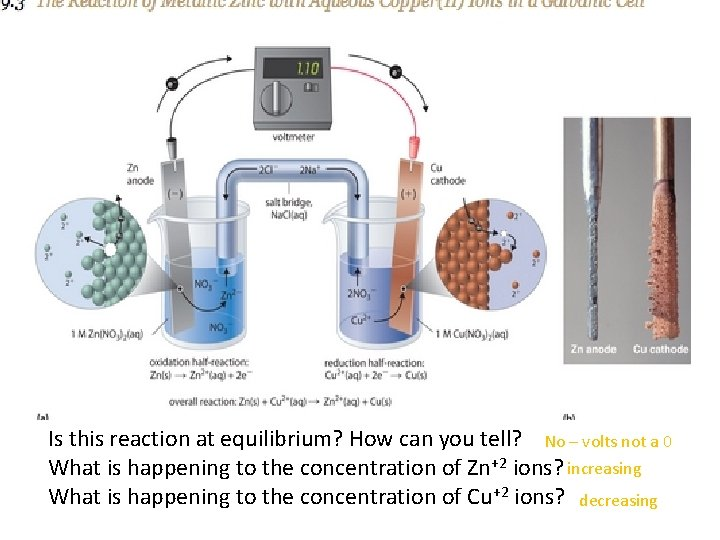Is this reaction at equilibrium? How can you tell? No – volts not a 0 What is happening to the concentration of Zn+2 ions? increasing What is happening to the concentration of Cu+2 ions? decreasing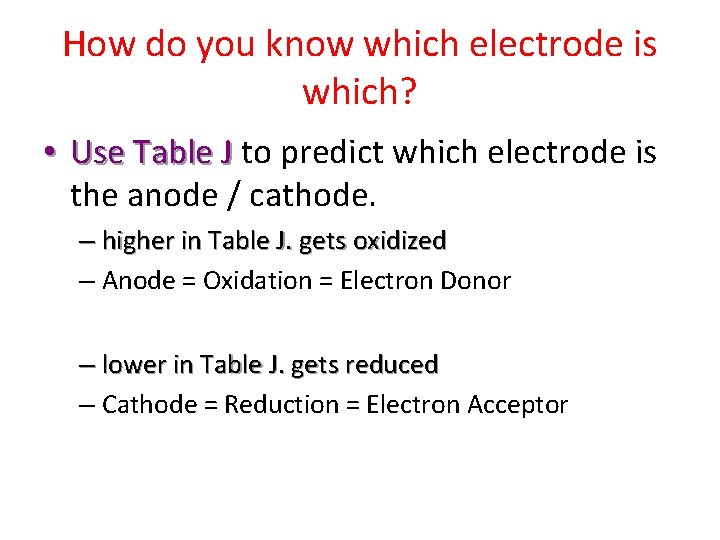How do you know which electrode is which? • Use Table J to predict which electrode is the anode / cathode. – higher in Table J. gets oxidized – Anode = Oxidation = Electron Donor – lower in Table J. gets reduced – Cathode = Reduction = Electron AcceptorLabel anode and cathode anode cathode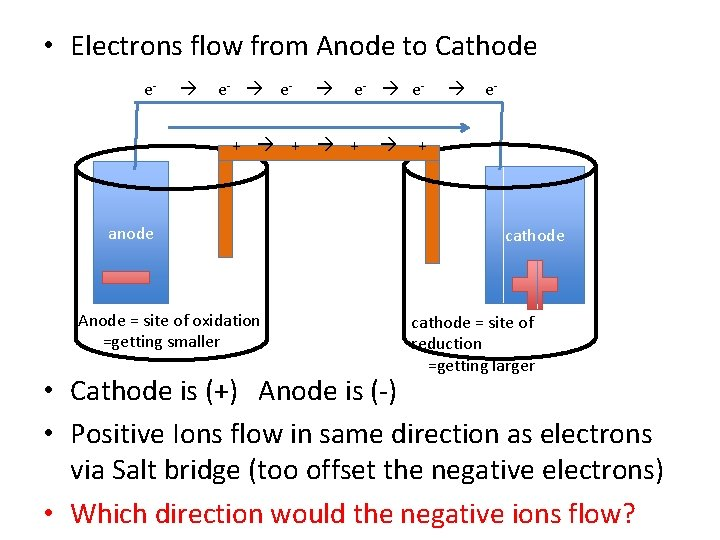• Electrons flow from Anode to Cathode e- e- e- + + + anode Anode = site of oxidation =getting smaller e- + cathode = site of reduction =getting larger • Cathode is (+) Anode is (-) • Positive Ions flow in same direction as electrons via Salt bridge (too offset the negative electrons) • Which direction would the negative ions flow?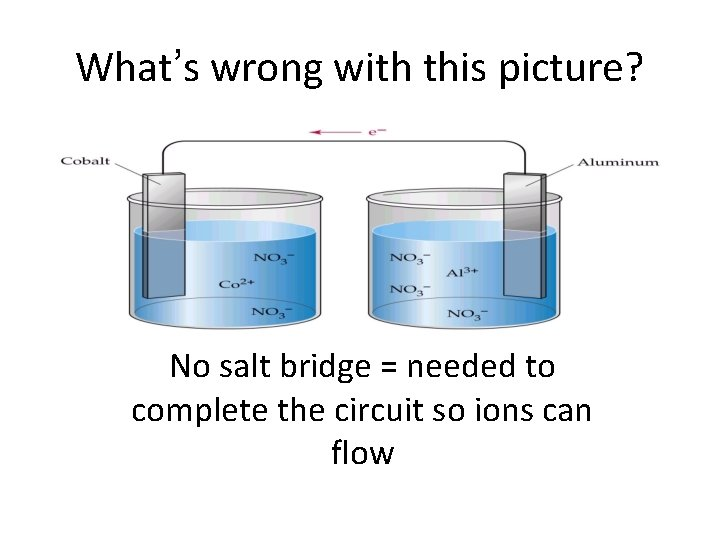What’s wrong with this picture? No salt bridge = needed to complete the circuit so ions can flowNow you try Create an galvanic (electrochemical) cell with Al and Pb and Al. NO 3 and Pb(NO 3)2 Label anode and cathode(Use Table J), direction of electron flow in wire, direction of positive ion flow in salt bridge, positive electrode, negative electrode. Pb Al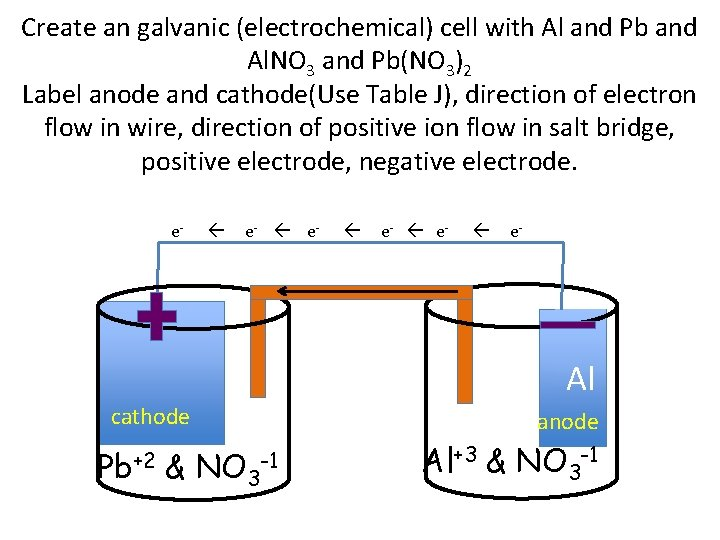Create an galvanic (electrochemical) cell with Al and Pb and Al. NO 3 and Pb(NO 3)2 Label anode and cathode(Use Table J), direction of electron flow in wire, direction of positive ion flow in salt bridge, positive electrode, negative electrode. e- e- Pb & NO 3 e- e- Al Al cathode Pb+2 anode -1 Al+3 & NO 3 -1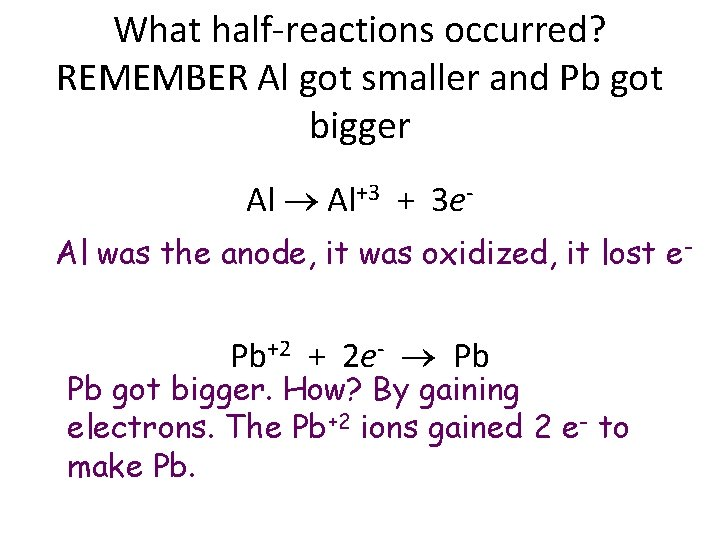What half-reactions occurred? REMEMBER Al got smaller and Pb got bigger Al Al+3 + 3 e. Al was the anode, it was oxidized, it lost e- Pb+2 + 2 e- Pb Pb got bigger. How? By gaining electrons. The Pb+2 ions gained 2 e- to make Pb.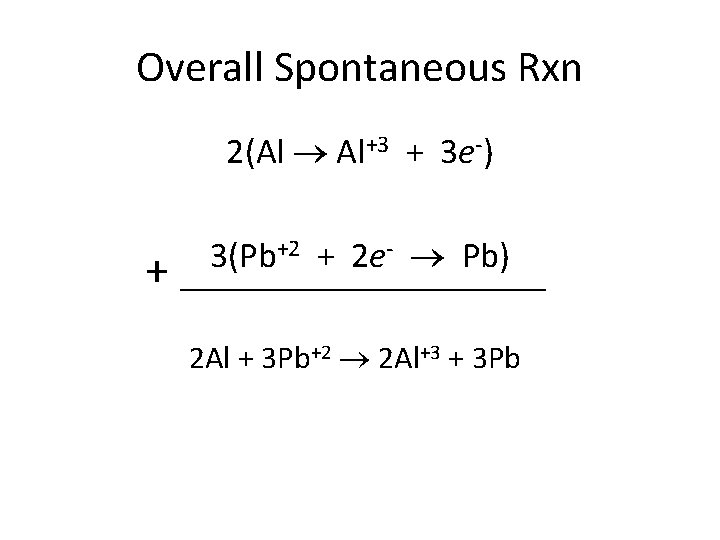Overall Spontaneous Rxn 2(Al Al+3 + 3 e-) + +2 + 2 e- Pb) 3(Pb ____________ 2 Al + 3 Pb+2 2 Al+3 + 3 Pb2 Al + 3 Pb+2 2 Al+3 + 3 Pb • Which electrode is losing mass? Al because now Al+3 ions in solution • Which electrode is gaining mass? Pb, Pb+2 gains 2 e- an makes solid Pb • What’s happening to the [Al+3]? Increasing • What’s happening to the [Pb+2]? DecreasingNotation for CellsApplication: BatteriesDry Cell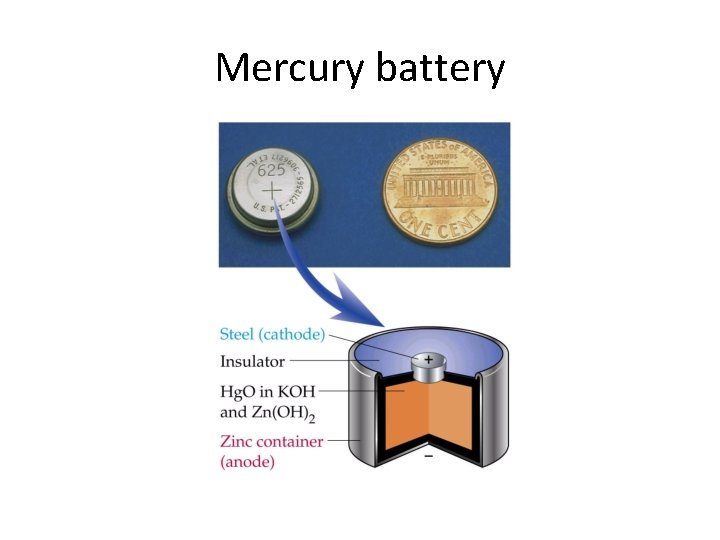Mercury battery